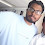# Pharma Engineering

For Engineer By Engineer

• ## Sunday, 17 February 2019

I'm back with a post today after a long gap.
Being busy with regular job role, can't be that much active here.

Today i gonna deliver about the FBD (Fluidised Bed Dryer) and i'll let you know why i've selected this. In the recent days i've worked on calculating the required blower capacity and evaluated many things related to FBD working.

And before explaining the way of calculating the blower capacity, its quite necessary to know about these.
Whats the working principle of FBD ?
FBD works on the principle of Fluidisation.

What is the mode of heat transfer in FBD ?
Convective heat transfer with direct contact mode.

What are the main components of FBD ?
Main components of FBD are filter bags, collection bowl, filters, blower, fluid heating unit.

What sort of filter bags used in FBD ?

Finger bags

What should be the blower capacity ?
Blower capacity will vary and it is based on the particle size and material density.

What is fluidization velocity ?

Minimum fluid velocity required to lift the particles(Simply fluidize) upto the finger bags.
Lets start the calculation part by assuming a case.

Lets suppose, we need to fluidise a material containing particles with density of 0.3 Kg/m3(on an average) with air and lets say the particle size be around 250 µm. And let say the void fraction between the particles be 0.3(this is the assumption i made). Let the duct diameter of the FBD be 12".

Hope you have an idea on the FBD constriction. If not, pl find it below:
From the above, you can find the duct of the FBD.

Now lets complete calculation for the calculation. For that we need to know some basic calculations again.

1. Surface area of the particle (As): 4𝚷 r^2 = 4 x 3.141 x (250/2)^2 / (10^6)
= 0.20 µm2.
You may get a doubt, that from where shall we get the particle size, that's an easy task actually, consider the d(0.9) from the PSD(Particle Size Distribution) report. Here in this case i've considered the particle as spherical.

2. Volume of the particle (V): (4/3) 𝚷 r^3 = (4/3) x 3.141 x (250/2)^2/(10^12)
= 8.18 x 10^-6 µm3

3. Sphericity of the particle: ( 6 / Dp) / ( As / V ) = (6/250)/(0.2/8.18 x 10^-6)
= 1.00 x 10^-6.

4. Viscosity of the fluid : There is no calculation for this, as we are fluidizing with air, we'll consider as 18.1 µPa.

5. Density of fluid: density of air = [(0.79 x 28) + (0.21 x 32)] / 22.414 = 1.29 Kg/m3.

0.79 - Nitrogen composition in air, 0.21 - Oxygen composition in air.
28 - Nitrogen molecular weight, 32 - Oxygen molecular weight.
22.414 - At STP, one mole of gas occupies 22.414 L of volume.
Now keep your cool, i gonna show you a formula, find it below:

Here, the Umf represents the fluidization velocity.
ϵmf is the void space and remaining all you know.

By subjecting the Umf, we will get a typical equation like this, below is the equation i derived:
1.75 (Umf^2)  +  5.89x10^6 (Umf) + 0.00 = 0

For this we have to derive the roots by using our +2 brains,

The roots shall be 0, -3.37 x 10^6.

If you don't remember the formula, pl check it,

one root will be: [-b + SQRT(b^2 - 4ac)]/2a,
second root will be: [-b - SQRT(b^2 - 4ac)]/2a

So, in my case i got two roots, one is positive and another is negative,
As the FBD runs through blower suction and suction means -Ve,
i'll consider only the negative value not the positive value.

You may get a doubt 😅that, what if we get two roots as negative, then in that case consider the lower value(for better results).

So here the fluidization velocity is found to be 3.37 x 10^6 µm/sec.

[Just remember, if any conversions went wrong,
then the result would be the velocity of Tsunami]

Now required blower capacity shall be calculated based on our engineering brains, as

Flow rate = Velocity x Area of cross section.

I think, now you got an idea why i've mentioned the duct area 😅

Blower capacity required = 𝛑 r^2 x Vs = (3.141 x (250/2)^2) x (3.37 x 10^6)
=  884 m3/hr

And i'll consider only 80% efficiency, so it will be

Total capacity required = 884 / 0.8 = 1105 m3/hr = 651 CFM.

That's it........!!! You are done.

Hope you got it..........!!!

If any queries feel free comment / message me........!!!

Jus remember, comments are most appreciated..................!!!!

Related Posts:
[How to] Design a decanter for workups in Pharma ?
Agitated Thin Film Dryer Design calculations
[How to] Calculate vapour column diameter ?
[How to] Perform Scale-Up for process equipment's ?

** This post is under construction since three weeks and got some time today to finish this.

And i would like to add something here, recently i received a mail from a guy and he is claiming that the content i'm posting was scrapped from somewhere and for those guys i would like to say proudly that,

"I'm an engineer and i would like to develop some purposeful content, instead of Ctrl+C and Ctrl+V".Hi! I am Ajay Kumar Kalva, Currently serving as the CEO of this site, a tech geek by passion, and a chemical process engineer by profession, i'm interested in writing articles regarding technology, hacking and pharma technology.

1.Thank you so much sir, your contents are very insightful. Sir if possible then can you post something on ejector (steam and water) both and calculation involved in it.

1.Dear Blesson,

Thanks, and i'll post it soon

Regards,
AJAY K

## This Blog is protected by DMCA.comHi! I am Ajay Kumar Kalva, owner of this site, a tech geek by passion, and a chemical process engineer by profession, i'm interested in writing articles regarding technology, hacking and pharma technology.